# Optimal decoding

(diff) ← Older revision | Latest revision (diff) | Newer revision → (diff)

A decoding which maximizes the exactness of information reproduction (cf. Information, exactness of reproducibility of) for given sources of information, communication channels and coding methods. In case the exactness of information reproduction is characterized by the mean probability of erroneous decoding (cf. Erroneous decoding, probability of), optimal decoding minimizes this probability. For example, for a transmissionof information represented by the numbers, the probabilities of appearance of which are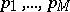, respectively, a discrete channel is used with a finite number of input and output signals and with transition function defined by the matrix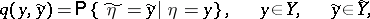where,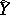are the sets of values of the signals of the inputand the output, respectively, while the coding is defined by the functionfor which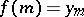,, where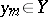,, is a code, i.e. a choice ofpossible input values. Then an optimal decoding is defined by a function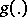such thatfor any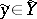, wheresatisfies the inequality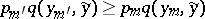for all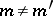. In particular, if all information has equal probability, i.e., then the described optimal decoding is also a decoding by the method of "maximum likelihood" (which is generally not optimal): The output signal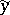has to be decoded in the information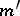for which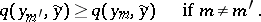How to Cite This Entry:
Optimal decoding. Encyclopedia of Mathematics. URL: http://encyclopediaofmath.org/index.php?title=Optimal_decoding&oldid=12294
This article was adapted from an original article by R.L. DobrushinV.V. Prelov (originator), which appeared in Encyclopedia of Mathematics - ISBN 1402006098. See original article